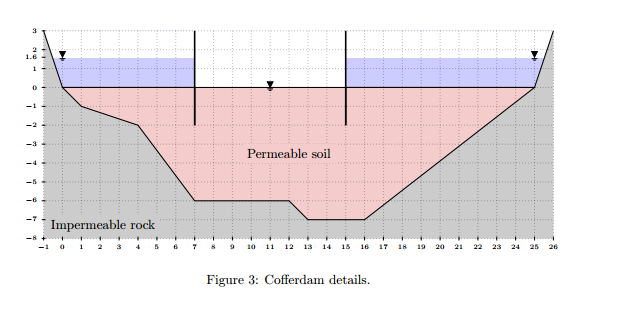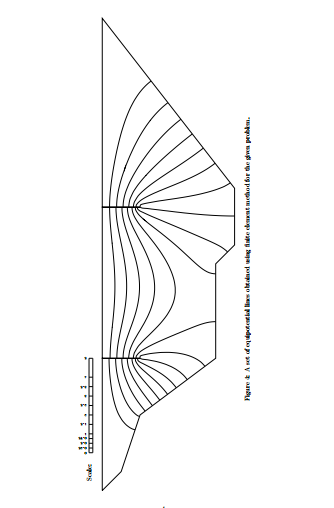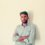# Need the Solution!A simple cofferdam is constructed by installing two long parallel sheetpiles in a river bed as shown in Fig 3. Dimensions are in meters. Between the two sheetpiles, water will be pumped out to prepare an area for construction of a bridge column. Outside the cofferdam, the water level is estimated to be 1.6 m. A seepage analysis is necessary to find out the required pumping capacity and also to assess the stability of the sheetpiles. A numerical analysis using finite element method is conducted and a group of contour lines for different pressure heads (equipotential lines) within the permeable area are calculated and reported in Fig 4.

Requirements
You need to use this preliminary numerical result to:
B1. Complete the flow net.
B2. Calculate the seepage rate and the required pumping capacity [Volume/Time] for every meter
of the cofferdam. Assume a hydraulic conductivity of k = 0.027 mm/sec for the soil.
B3. Sketch the water pressure distribution on both sides of both sheet piles.
B4. Calculate the magnitude and location of the resultant force caused by water pressure on both
sides of both sheet piles. You need to calculate the location and magnitude of one force for
each side of each sheetpile (i.e. four forces altogether).Note by Mehdi Balti
5 years, 10 months ago

This discussion board is a place to discuss our Daily Challenges and the math and science related to those challenges. Explanations are more than just a solution — they should explain the steps and thinking strategies that you used to obtain the solution. Comments should further the discussion of math and science.

When posting on Brilliant:

• Use the emojis to react to an explanation, whether you're congratulating a job well done , or just really confused .
• Ask specific questions about the challenge or the steps in somebody's explanation. Well-posed questions can add a lot to the discussion, but posting "I don't understand!" doesn't help anyone.
• Try to contribute something new to the discussion, whether it is an extension, generalization or other idea related to the challenge.

MarkdownAppears as
*italics* or _italics_ italics
**bold** or __bold__ bold
- bulleted- list
• bulleted
• list
1. numbered2. list
1. numbered
2. list
Note: you must add a full line of space before and after lists for them to show up correctly
paragraph 1paragraph 2

paragraph 1

paragraph 2

[example link](https://brilliant.org)example link
> This is a quote
This is a quote
    # I indented these lines
# 4 spaces, and now they show
# up as a code block.

print "hello world"
# I indented these lines
# 4 spaces, and now they show
# up as a code block.

print "hello world"
MathAppears as
Remember to wrap math in $$ ... $$ or $ ... $ to ensure proper formatting.
2 \times 3 $2 \times 3$
2^{34} $2^{34}$
a_{i-1} $a_{i-1}$
\frac{2}{3} $\frac{2}{3}$
\sqrt{2} $\sqrt{2}$
\sum_{i=1}^3 $\sum_{i=1}^3$
\sin \theta $\sin \theta$
\boxed{123} $\boxed{123}$

Sort by:

What have you tried? What are your thoughts?

Staff - 5 years, 10 months ago